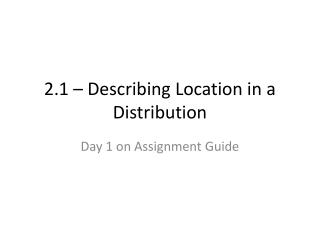# 2.1 – Describing Location in a Distribution - PowerPoint PPT PresentationDownload Presentation2.1 – Describing Location in a Distribution

2.1 – Describing Location in a Distribution
Download Presentation## 2.1 – Describing Location in a Distribution

- - - - - - - - - - - - - - - - - - - - - - - - - - - E N D - - - - - - - - - - - - - - - - - - - - - - - - - - -
##### Presentation Transcript

1. 2.1 – Describing Location in a Distribution Day 1 on Assignment Guide

2. Percentiles • The percent of observations in a set of data that are below a certain value. • It is possible to include equal to, but we will strictly do below for consistency

3. Cumulative Relative Frequency Plot • Remember relative frequency is percent • Cumulative – running total AKA “Ogives”

4. Shape for Cumulative Relative Frequency Plot Where is the graph the steepest? What does this mean? Sketch a Cumulative Relative Frequency Plot for this data. Describe the shape. Sketch a Cumulative Relative Frequency Plot for symmetric and skewed left

5. Check Your Understanding Turn to page 89 and do problems 1 – 4 We will do one at a time on the whiteboards

6. z-scores • Also called standardized value • It is unit-less • Tells us how many standard deviations away from the mean a particular value is • Formula is on your formula sheet and on p. 90

7. In 2009, the mean number of wins in MLB was 81 with a standard deviation of 11.4 wins. • Find and interpret the z-score for the NY Yankees if they had 103 wins. • Find and interpret the z-score for the NY Mets if they had 70 wins. z-score = (103-81)/11.4 = 1.93. Interpret: The NY Yankees were 1.93 standard deviations above the mean number of wins. z-score = (70-81)/11.4 = -0.96. Interpret: The NY Mets were 0.96 standard deviations below the mean number of wins.